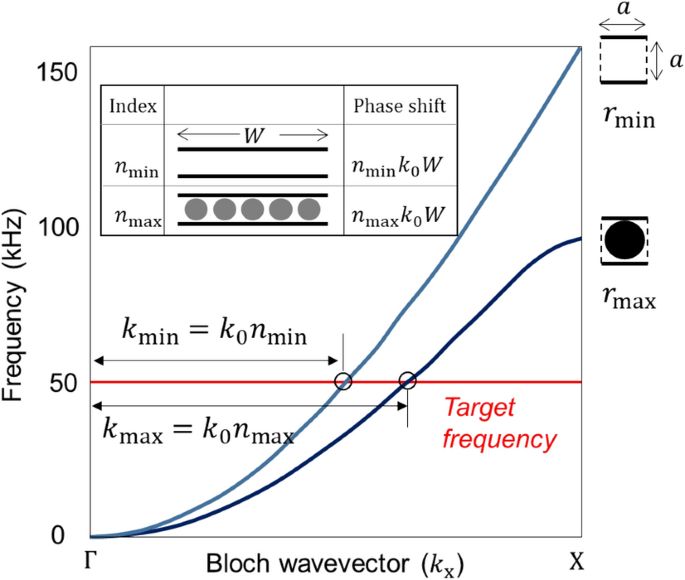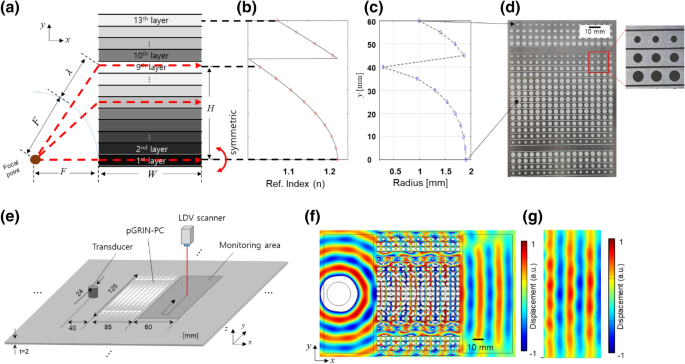# Partitioned gradient-index phononic crystals for full phase control[ad_1]

### Partitioned gradient-index phononic crystals

In order to link and control the phase at the transmitted end of the lens directly using the refractive indices in the gradient-index phononic crystal (GRIN-PC), we introduce partitions between the neighboring layers in the GRIN-PC system. By having extremely different impedance property from the medium (e.g., air in slits vs. aluminum plate), the “partitions” can prohibit the propagating waves in each layer from interfering with those in the neighboring layers. Note that a partition is often used for metasurfaces29 to divide neighboring sections as well, but this concept has not been used for a GRIN-PC system to the best of the authors’ knowledge. The partitioned GRIN-PC (pGRIN-PC) design allows the generalized Snell’s law, commonly used when designing metasurfaces2,4,22,24,25,26,27,28, for PC-designers to readily calculate the phase shifts based on the target wave modulation. Hereafter, we will use the term “phase shift” for the phase difference between the incident- and the transmitted- sides of the lens and “phase difference” for the others such as difference between phases at the transmitted (right-hand-side) end of a layer and at the same end of the center layer (or the 1st layer) of a flat lens.

Figure 1a,b show the pGRIN-PC system and its conventional counterpart without a partition, respectively, with each layer containing five unit cells in both systems. In pGRIN-PC, the square unit cell consists of a hole with radius r in the center and partitions on the upper and lower sides on an aluminum plate with a thickness of 2 mm, as shown in Fig. 1a. These partitions guide the incident wave, causing it to propagate only in the x direction, while Fig. 1b shows the wave paths are bent due to the index difference in the neighboring layers of the conventional GRIN-PC. Hence, the phase shift ({phi }_{mathrm{PC}}) in a layer between the incident and the transmitted sides (as indicated by the arrows in Fig. 1a) can be readily calculated via

$$phi_{{{text{PC}}}} = n_{{{text{PC}}}} k_{0} W,$$

(1)

where ({n}_{mathrm{PC}}) is the effective refractive index, ({k}_{0}) is the wavenumber of the surrounding medium, and W is the width of the pGRIN-PC. If we want a particular phase at the transmitted end of the lens, the corresponding indices can readily be computed by Eq. (1) for the pGRIN-PC case.Figure 1

### Systematic design process of pGRIN-PC systems

The entire design process to model the pGRIN-PC consists of three steps: (1) setting the refractive index range, (2) calculating the width to cover a full phase from 0 to 2π, and (3) finding the inclusion radii in the layers for target purposes depending on the design, here, a collimator. It would be ideal for the partition to have a width of zero; however, due to manufacturability issues, a through-cut with a 0.5 mm width is chosen instead, and thus the through-cut line of 0.5 mm widths are utilized as the partitions. Accordingly, the sizes of the upper and lower partitions in one unit cell are both 0.25 mm. The target frequency is 50 kHz, and the corresponding wavelength (uplambda ) is approximately 18.7 mm for flexural wave (A0 Lamb wave). The size of the unit cell, a, is set to 5 mm ((simuplambda /4)).

First, in order to find the index range, the minimum and maximum indices are computed. Because the radius r is the only parameter to be controlled in the index design, the minimum and the maximum radii of the unit cell define the index range applied to the pGRIN-PC lens. It is reasonable to set the minimum radius to zero, whereas the maximum value is set to the largest achievable radius of 1.9 mm, considering manufacturability, and the corresponding unit cells are shown in Fig. 2. Note that these values can differ depending on the PC and the environment. Once the two radii are selected, the corresponding effective wavenumbers can be, for example, obtained from the band structures computed by COMSOL multiphysics, as shown in Fig. 2. Then, their effective refractive indices at the target frequency can be calculated using the equation

$$n_{{{text{PC}}}} = k_{{{text{PC}}}} /k_{0} ,$$

(2)

where ({k}_{mathrm{PC}}) is the effective wavenumber of the unit cell. At 50 kHz, the wavenumbers obtained for the radii 0 and 1.9 mm in Fig. 2 are 327.24 and 400.57 [1/m], respectively, and their refractive indices are 1 and 1.2237, which defines the limits for the index range.Figure 2

Second, it is necessary to calculate the width W to cover a full phase from 0 to 2π. Note that, in the pGRIN-PC case, the phase difference (Delta {phi }_{mathrm{min}}^{mathrm{max}}) resulting from unit cells with the two extreme radii are usually smaller than 2 (uppi ),(Delta {phi }_{mathrm{min}}^{mathrm{max}}=({n}_{mathrm{max}}-{n}_{mathrm{min}}){k}_{0}a<2pi ), because an index change in one unit cell of a phononic crystal is much smaller than, for example, that in resonance-based metamaterials. Therefore, one layer should consist of the number of unit cells that results in a 2π difference, and the width W of the layers becomes integer multiples of the unit cell size.

The width W of all layers in the pGRIN-PC system must be identical to the lens width. When waves propagate to a distance W, the difference of the phase shifts in the layers of the minimum and maximum refractive indices can be calculated as (({k}_{mathrm{max}}-{k}_{mathrm{min}})W), which should be 2 (uppi ) to cover the full phase. By comparing the difference and the refractive index in Eq. (2), the width W can be expressed as:

$$W = frac{lambda }{{n_{{max}} – n_{{min}} }},$$

(3)

where (lambda =2uppi /{mathrm{k}}_{0}) is the wavelength in the surrounding medium. The width obtained from Eq. (3) is 83.5 mm for the collimator, and the layer is selected to have 17 unit cells; thus, the layer width is expressed as W = 85 mm (= 17 unit cells × 5 mm/unit cell), as close as possible to 83.5 mm.

The third step is to find the indices for the layers other than the two layers with the minimum and maximum radii and then to determine their corresponding geometrical parameters, i.e., the hole radius r. Note that the three-step design methodology is utilized mainly for designing phononic crystals, although larger in size, which operates in a wider frequency range than the metamaterials30. In the pGRIN-PC case, the indices are readily found when the target wave directions are set, whereas designing such a conventional GRIN-PC system with the same profile requires tremendous computational labor to find them. Once the indices are selected, finding the corresponding radii is an inverse design process. In order to find the shape and size of the inclusion for the target refractive index, a number of methods can be used, such as trial/error31, optimization29,32,33,34 and machine learning35. In this paper, we derive a fifth-order polynomial to link the refractive indices to the radii and use it to find the hole radii. The unit cell of a GRIN-PC lens often has a simple inclusion geometry, as shown in Fig. 1, and a polynomial for such a shape can readily be found with fewer sample points than the number required for the trial/error method31.

In order to derive the equation, ten different radius values between 0.3 to 1.9 mm were selected, and their refractive indices were computed by Eq. (2) with the effective wavenumbers from the band structures, as indicated in Fig. 2. From the ten radius-index relationships, we derive a fifth-order polynomial by means of the least square method:

$$10^{ – 4} r = 1.35n^{5} – 7.79n^{4} + 17.95n^{3} – 20.66n^{2} + 11.89n – 2.74$$

(4)

Note that Eq. (4) can be used for designing not only for the collimator example but also for a pGRIN-PC system for any wave modulation, such as the Bessel beam or focusing types. Provided the refractive indices, we are then able to find the radius sizes with the equation.

### Applications of pGRIN-PC and experimental realization

As a representative example, a pGRIN-PC based collimator is designed to create a plane wave from a cylindrical source. For the collimator design, phase shift from the source point to the right-side of all layers in the pGRIN-PC must be equal as the red dashed-lines in Fig. 3a, from which the refractive indices of the layers can be calculated as in Fig. 3b. The corresponding radii for the layers in the pGRIN-PC system is computed by Eq. (4) as indicated in Fig. 3c (detailed equations are presented in the supplementary notes). Note that the refractive index in Fig. 3b shows an abrupt jump between the 9th and 10th layers, since the target phase required for the layers above the 10th layer are larger than 2 (uppi ), in which case we reset the target phase to be remainder of the original one divided by (2uppi ).Figure 3

The designed pGRIN-PC for the collimator is then manufactured in Fig. 3d and experimentally characterized for validation. The experimental setup is shown in Fig. 3e. A transducer with a 50 kHz resonance frequency is attached to the plate, and a laser Doppler vibrometer (LDV) scans the flexural displacement normal to the plate. Note that a rather large (1.8 mtimes 1.2 m) aluminum plate is used for the pGRIN-PC being manufactured on in order to separate the unwanted waves reflected from the plate boundaries and the waves of interest transmitted from the pGRIN-PC. Detailed descriptions of this setup are provided in the supplementary note. In parallel with the experiments, harmonic simulations are conducted using COMSOL Multiphysics. In Fig. 3f,g, the simulation and the experimental results are in good agreement, showing that the outgoing waves are in-phase. The large radius difference between the 9th and the 10th layers as in Fig. 3c seems to lessen the lens’ performance due to the impedance mismatch, which can be witnessed in Fig. 3f, but enhancing the lens’ quality will be left for the future work. Nevertheless, the plane wave is clearly shown to propagate in Fig. 3f,g, demonstrating that the wave is successfully collimated. In order to ensure the full phase controllability of the proposed pGRIN-PC for the collimator, we performed additional experiment where focusing is observed upon the incident waves on the collimators Fig. S7, which is fully described in the Supplementary Note.

In addition, in order to illustrate the further applicability of the pGRIN-PC system, we applied our pGRIN-PC design methodology to another example, in this case, a Bessel beam generator. A Bessel beam is a beam with a high aspect-ratio beam size. It can be created in theory by two plane waves directing opposite angles (beta ) and (-beta ), implying that the wave along the mirror axis does not spread out.

The unit cell configuration for this example is selected to be identical to that used for the collimator design, as shown in Fig. 1a. Thus, if the target refractive index for the Bessel beam generator is chosen, its target radii are readily found using Eq. (4). The Bessel beam in this paper is designed to have a refractive angle of (beta ={20}^{circ }), and the indices of the layers increase linearly from the center layer in order to create two plane waves directing opposite angles ({pm 20}^{circ }). In this Bessel beam case, we set the maximum radius to 1.66 mm, different from the collimator case, in order to show that the pGRIN-PC system can be built with other index ranges when using the proposed design methodology. In this case, the corresponding index is 1.16, the width is then calculated and found to be 116.8 mm, and thus W is set to have 24 unit cells such that 24 ea. × 5 mm = 120 mm.

Figure 4a presents the experimental setup for the Bessel-beam generator, and Fig. 4b shows the fabricated Bessel beam generator based on the pGRIN-PC design. The simulation result in Fig. 4c clearly visualizes the function of the Bessel beam generator with the targeted ({20}^{circ }) angle. Figure 4d experimentally confirms the performance of the Bessel beam generator, which is also in good agreement with the simulated results in Fig. 4c given the ({20}^{circ }) refraction angles.Figure 4

[ad_2]

Source link